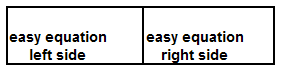The Visual / Auditory / Symbolic / Kinesthetic Approach to Algebra

 Solve A Systems of Linear Equations by Substitution.

Example 3a. in the Warm Ups, from the last page and just below, is a SYSTEM of linear equations presented as a SINGLE sophisticated linear equation. To solve it, substitution is employed. Below, examine it now from a linear system perspective.Solve Linear Systems by Substitution.1st: Use two display areas side-by-side for representing an equation. (These will later be moved to the top away from the user.) 2nd: Pick one equation to solve for one variable. Take the easy equation and the easy variable or use another method. 3rd: Solve the equation for the variable of choice. 4th: Move the solved equation in the side-by-side display areas to the top, away from the user, and use two additional side-by-side display areas for the other equation, placing them on the bottom close to the user.5th: Represent the other equation IN THE STORAGE AREAS ABOVE THE DISPLAY AREAS. 6th: In the bottom display areas, SUBSTITUTE, replace, each of the solved variable tiles with the expression found in the third step and duplicate exactly all other tiles. 7th: Solve the equation from the step six. 8th: Move the easy equation to the top storage areas above the display areas.9th: In the top display areas, SUBSTITUTE, replace, each of the other variable tiles with the new value from step 7 and duplicate exactly all other tiles. 10th: Solve the easy equation. 11th: State the solution and include values for both variables.www.termtiles.com, Unit 38   © 2008, A. Azzolino